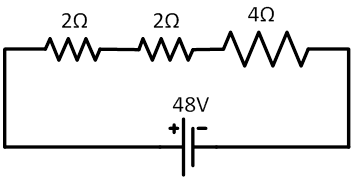### Sample ProblemWhat is the total current in this circuit? What is the current for each individual

resistor in the circuit?

#### Solution

Using the equation V = IR, we plug in our given values (8Ω is the total resistance, since resistors add in series) and get 48 = 8I, I = 6A. We know that current is the same across series resistors.